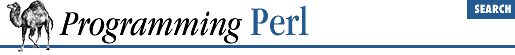home | O'Reilly's CD bookshelfs | FreeBSD | Linux | Cisco | Cisco Exam

#### 7.2.42 Math::BigFloat - Arbitrary-Length, Floating-Point Math Package

```use Math::BigFloat;

\$f = Math::BigFloat->new(\$string);

# ```

NSTR
```
is a number string; ```

SCALE
```
is an integer value.
# In all following cases \$f remains unchanged.
# All methods except fcmp() return a number string.
\$f->fadd(```

NSTR
```
);          # return sum of ```

NSTR
```
and \$f
\$f->fsub(```

NSTR
```
);          # return \$f minus ```

NSTR
```

\$f->fmul(```

NSTR
```
);          # return \$f multiplied by ```

NSTR
```

\$f->fdiv(```

NSTR
```
[,```

SCALE
```
]);  # return \$f divided by ```

NSTR
```
to ```

SCALE
```
places
\$f->fneg();              # return negative of \$f
\$f->fabs();              # return absolute value of \$f
\$f->fcmp(```

NSTR
```
);          # compare \$f to ```

NSTR
```
; see below for return value
\$f->fround(```

SCALE
```
);       # return rounded value of \$f to ```

SCALE
```
digits
\$f->ffround(```

SCALE
```
);      # return rounded value of \$f at ```

SCALEth
```
place
\$f->fnorm();             # return normalization of \$f
\$f->fsqrt([```

SCALE
```
]);      # return sqrt of \$f to ```

SCALE
```
places```

This module allows you to use floating-point numbers of arbitrary length. For example:

`\$float = new Math::BigFloat "2.123123123123123123123123123123123";`

Number strings (``` NSTR ``` s) have the form, ``` /[+-]\d*\.?\d*E[+-]\d+/``` . Embedded white space is ignored, so that the number strings used in the following two lines are identical:

```\$f = Math::BigFloat->new("-20.0    0732");
\$g = \$f->fmul("-20.00732");```

The return value ``` NaN``` indicates either that an input parameter was "Not a Number", or else that you tried to divide by zero or take the square root of a negative number. The ``` fcmp()``` method returns ``` -1``` , ``` 0``` , or ``` 1``` depending on whether ``` \$f``` is less than, equal to, or greater than the number string given as an argument. If the number string is undefined or null, the undefined value is returned.

If ``` SCALE ``` is unspecified, division is computed to the number of digits given by:

`max(\$div_scale, length(dividend)+length(divisor))`

A similar default scale value is computed for square roots.

When you use this module, Perl's basic math operations are overloaded with routines from Math::BigFloat. Therefore, you don't have to employ the methods shown above to multiply, divide, and so on. You can rely instead on the usual operators. Given this code:

```\$f = Math::BigFloat->new("20.00732");
\$g = Math::BigFloat->new("1.7");```

the following six lines all yield the corresponding values for ``` \$h``` :

```\$h = 20.00732 * 1.7;    # 34.012444 (ordinary math--\$h is not an object)
\$h = \$f * \$g;           # "34.012444" (\$h is now a BigFloat object)
\$h = \$f * 1.7;          # "34.012444" (\$h is now a BigFloat object)
\$h = 20.00732 * \$g;     # "34.012444" (\$h is now a BigFloat object)
\$h = \$f->fmul(\$g);      # "+34012444E-6" (\$h is now a BigFloat object)
\$h = \$f->fmul(1.7);     # "+34012444E-6" (\$h is now a BigFloat object)```7.2.41 lib - Manipulate @INC at Compile-Time7.2.43 Math::BigInt - Arbitrary-Length Integer Math Package